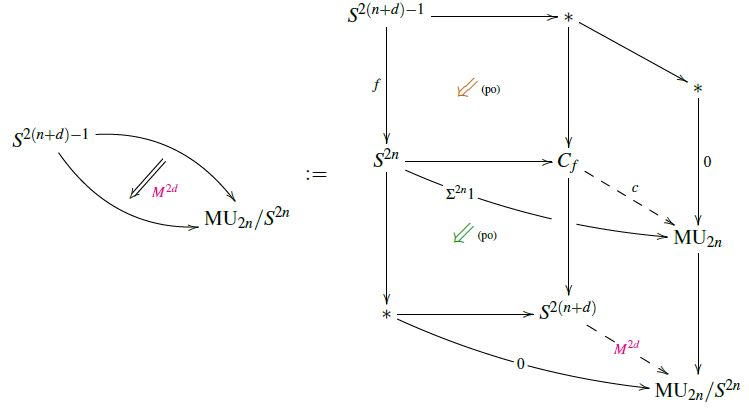# nLab e-invariant is Todd class of cobounding (U,fr)-manifold

Contents

### Context

#### Cobordism theory

Concepts of cobordism theory

flavors of bordism homology theories/cobordism cohomology theories, their representing Thom spectra and cobordism rings:

bordism theory$\;$M(B,f) (B-bordism):

relative bordism theories:

algebraic:

# Contents

## Idea

The e-invariant is, a priori, a homotopy invariant of morphisms in the stable homotopy category, hence in particular of the stable homotopy groups of spheres. Under the identification of the latter, via the Pontrjagin-Thom isomorphism, with the framed bordism ring, the e-invariant turns out to equal the Todd class of any cobounding (U,fr)-manifold (Conner-Floyd 66).

## Preliminaries

###### Remark

In generalization to how the U-bordism ring $\Omega^U_{2k}$ is represented by homotopy classes of maps into the Thom spectrum MU, so the (U,fr)-bordism ring $\Omega^{U,fr}_{2k}$ is represented by maps into the quotient spaces $MU_{2k}/S^{2k}$ (for $S^{2k} = Th(\mathbb{C}^{k}) \to Th( \mathbb{C}^k \times_{U(k)} E U(k) ) = MU_{2k}$ the canonical inclusion):

(1)$\Omega^{(U,fr)}_\bullet \;=\; \pi_{\bullet + 2k} \big( MU_{2k}/S^{2k} \big) \,, \;\;\;\;\; \text{for any} \; 2k \geq \bullet + 2 \,.$
###### Remark

The bordism rings for MU, MUFr and MFr sit in a short exact sequence of the form

(2)$0 \to \Omega^U_{\bullet+1} \overset{i}{\longrightarrow} \Omega^{U,fr}_{\bullet+1} \overset{\partial}{ \longrightarrow } \Omega^{fr}_\bullet \to 0 \,,$

where $i$ is the evident inclusion, while $\partial$ is restriction to the boundary.

(By this Prop. at MUFr.)

In particular, this means that $\partial$ is surjective, hence that every $Fr$-manifold is the boundary of a (U,fr)-manifold.

## Statement

###### Proposition

(Todd class on cobounding (U,fr)-manifolds computes e-invariant)

Evaluation of the Todd class on (U,fr)-manifolds yields rational numbers which are integers on actual $U$-manifolds. It follows with the short exact sequence (2) that assigning to $Fr$-manifolds the Todd class of any of their cobounding $(U,fr)$-manifolds yields a well-defined element in Q/Z.

Under the Pontrjagin-Thom isomorphism between the framed bordism ring and the stable homotopy group of spheres $\pi^s_\bullet$, this assignment coincides with the Adams e-invariant in its Q/Z-incarnation:

(3)$\array{ 0 \to & \Omega^U_{\bullet+1} & \overset{i}{\longrightarrow} & \Omega^{U,fr}_{\bullet+1} & \overset{\partial}{ \longrightarrow } & \Omega^{fr}_\bullet & \simeq & \pi^s_\bullet \\ & \big\downarrow{}^{\mathrlap{Td}} && \big\downarrow{}^{\mathrlap{Td}} && \big\downarrow{}^{} && \big\downarrow{}^{e_{\mathbb{C}}} \\ 0 \to & \mathbb{Z} &\overset{\;\;\;\;\;}{\hookrightarrow}& \mathbb{Q} &\overset{\;\;\;\;}{\longrightarrow}& \mathbb{Q}/\mathbb{Z} &=& \mathbb{Q}/\mathbb{Z} } \,,$
###### Proof

The first step in the proof of (3) is the observation (Conner-Floyd 66, p. 100-101) that the representing map (1) for a (U,fr)-manifold $M^{2k}$ cobounding a $Fr$-manifold represented by a map $f$ is given by the following homotopy pasting diagram (see also at Hopf invariantIn generalized cohomology):from SS21

From this, Conner-Floyd conclude essentially by considering the following homotopy pasting diagram (the diagrammatic perspective here follows SS21, for more see at Adams e-invariant via unit cofiber theories):

Here for the identification of the Todd class $Td$ in the bottom of the diagram we used that the rational Todd class is the Chern character of the Thom class.

That this Todd class equals the Q/Z-valued e-invariant follows now since the latter is the top-degree component of the Chern character of any complex topological K-theory-class $V_{2n} \coloneqq \sigma \circ c$ lifting $\Sigma^{2n} 1 \in \widetilde K(S^{2n})$ to $C_f$ (this Prop.).

## Variants

An analogous but finer construction works for special unitary group-structure instead of unitary group-structure and in dimensions $8\bullet + 4$:

Since on $(8 \bullet + 4)$-dimensional $SU$-manifolds the Todd class is divisible by 2 (Conner-Floyd 66, Prop. 16.4) we have (Conner-Floyd 66, p. 104) the following short exact sequence of MSUFr-bordism rings, in variation of (3):

(4)$\array{ 0 \to & \Omega^{SU}_{8\bullet+4} & \overset{i}{\longrightarrow} & \Omega^{SU,fr}_{8\bullet+4} & \overset{\partial}{ \longrightarrow } & \Omega^{fr}_{8\bullet + 3} & \simeq & \pi^s_{8\bullet + 3} \\ & \big\downarrow{}^{\tfrac{1}{2}\mathrlap{Td}} && \big\downarrow{}^{\tfrac{1}{2}\mathrlap{Td}} && \big\downarrow{}^{} && \big\downarrow{}^{e_{\mathbb{R}}} \\ 0 \to & \mathbb{Z} &\overset{\;\;\;\;\;}{\hookrightarrow}& \mathbb{Q} &\overset{\;\;\;\;}{\longrightarrow}& \mathbb{Q}/\mathbb{Z} &=& \mathbb{Q}/\mathbb{Z} } \,.$

This produces $e_{\mathbb{R}}$, the Adams e-invariant with respect to KO-theory instead of KU (Adams 66, p. 39), which, in degrees $8k + 3$, is indeed half of the e-invariant $e_{\mathbb{C}}$ for $KU$ (by Adams 66, Prop. 7.14).

In fact, for $k = 0$ we have:

###### Proposition

(Adams 66, Example 7.17 and p. 46)

In degree 3, the KO-theoretic e-invariant $e_{\mathbb{R}}$ takes the value $\left[\tfrac{1}{24}\right] \in \mathbb{Q}/\mathbb{Z}$ on the quaternionic Hopf fibration $S^7 \overset{h_{\mathbb{H}}}{\longrightarrow} S^4$ and hence reflects the full third stable homotopy group of spheres:

$\array{ \pi^s_3 & \underoverset{ \simeq }{ e_{\mathbb{R}} }{ \;\;\longrightarrow\;\; } & \mathbb{Z}/24 & \subset & \mathbb{Q}/\mathbb{Z} \\ [h_{\mathbb{H}}] &&\mapsto&& \left[\tfrac{1}{24}\right] }$

while $e_{\mathbb{C}}$ sees only “half” of it (by Adams 66, Prop. 7.14).

An alternative formulation via index theory:

Review in: